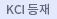다국어 입력

http://chineseinput.net/에서 pinyin(병음)방식으로 중국어를 변환할 수 있습니다.

변환된 중국어를 복사하여 사용하시면 됩니다.

예시)
• 中文 을 입력하시려면 zhongwen을 입력하시고 space를누르시면됩니다.
• 北京 을 입력하시려면 beijing을 입력하시고 space를 누르시면 됩니다.
닫기

검색결과 좁혀 보기

오늘 본 자료

• 오늘 본 자료가 없습니다.
더보기
• 무료
• 기관 내 무료
• 유료
•LNG 저장탱크 재액화용 LNG Sub-Cooler 및 N₂ Pre-Cooler 열전달 특성 및 구조안정성 해석

In this study, the heat transfer characteristics - in accordance with the operating conditions - of the heat exchanger for re-liquefaction of LNG storage tanks is analyzed. The heat transfer characteristics and structural stability were analyzed for each of the sub-cooler and pre-cooler, which uses an LNG fluid and an N2 fluid respectively. The N2 fluid is used in the actual experiment. Considering the efficiency and cost of the calculation, 1/25 and 1/49 reduced models were used for the number of pipes; the temperature difference among pipes at the outlet was less than 5%. Hence it was confirmed that the analysis of the reduced model was reasonable. Comparing with the one-dimensional analysis results, a discrepancy from 2.2% to 7.4% could be confirmed. By visualizing the flow field through the computational fluid cynamics (CFD) analysis, the cause of inefficiency could be identified. The analysis results will be helpful in understanding the exact performance of the heat exchanger by predicting the design variables in the future.